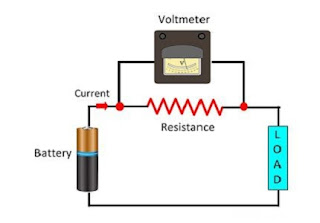## Page

### Why is Voltmeter connected in Parallel?

As shown in the figure, a voltmeter is connected in parallel with circuit elements because it is used to measure the voltage of the device. So important is that because elements in series have a high potential difference and high internal resistance. The amount of current passing through very less. Therefore the current through the circuit is unaltered.Parallel Connection

If the voltmeter is connected in series with the circuit, it will change the potential difference which will minimize the current in the circuit as it has very large resistance and you will get faulty reading. Thus the disturb the reading of the voltmeter.

As shown in the figure, a voltmeter is connected in parallel with circuit elements because it is used to measure the voltage of the device. So important is that because elements in series have a high potential difference and high internal resistance. The amount of current passing through very less. Therefore the current through the circuit is unaltered.Parallel Connection

If the voltmeter is connected in series with the circuit, it will change the potential difference which will minimize the current in the circuit as it has very large resistance and you will get faulty reading. Thus the disturb the reading of the voltmeter.